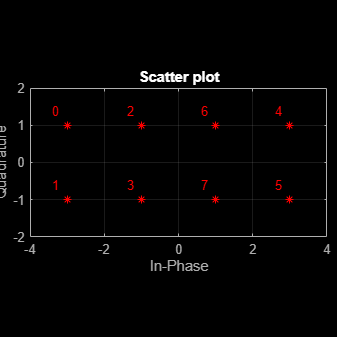## Plot Signal Constellations

### Create 16-PSK Constellation Diagram

This example shows how to plot a PSK constellation having 16 points.

Set the parameters for 16-PSK modulation with no phase offset and binary symbol mapping.

```M = 16; % Modulation alphabet size phOffset = 0; % Phase offset symMap = 'binary'; % Symbol mapping (either 'binary' or 'gray')```

Construct the modulator object.

`pskModulator = comm.PSKModulator(M,phOffset,'SymbolMapping',symMap);`

Plot the constellation.

`constellation(pskModulator)`### Create 32-QAM Constellation Diagram

This example shows how to plot a QAM constellation having 32 points.

Use the `qammod` function to generate the 32-QAM symbols with binary symbol ordering.

```M = 32; data = 0:M-1; sym = qammod(data,M,'bin');```

Plot the constellation. Label the order of the constellation symbols.

```scatterplot(sym,1,0,'b*'); for k = 1:M text(real(sym(k))-0.4,imag(sym(k))+0.4,num2str(data(k))); end axis([-6 6 -6 6])```### Create 8-QAM Gray Coded Constellation Diagram

Use the qammod function to generate the 8-QAM symbols with Gray symbol ordering. Note that Gray coding is the default symbol mapping for the `qammod` function.

```M = 8; data = 0:M-1; sym = qammod(data,M);```

Plot the constellation. Label the order of the constellation symbols.

```scatterplot(sym,1,0,'r*'); grid on for k = 1:M text(real(sym(k))-0.4,imag(sym(k))+0.4,num2str(data(k))); end axis([-4 4 -2 2])```### Plot a Triangular Constellation for QAM

This example shows how to plot a customized QAM reference constellation.

Describe the constellation.

```inphase = [1/2 -1/2 1 0 3/2 -3/2 1 -1]; quadr = [1 1 0 2 1 1 2 2]; inphase = [inphase; -inphase]; inphase = inphase(:); quadr = [quadr; -quadr]; quadr = quadr(:); refConst = inphase + 1i*quadr; ```

Construct a constellation diagram System object™ using name-value pairs to specify the title, the axes limits, the reference marker type, and the reference marker color.

```constDiagram = comm.ConstellationDiagram('Title','Customized Constellation for QAM', ... 'XLimits',[-3 3],'YLimits',[-3 3], ... 'ReferenceConstellation',refConst, ... 'ReferenceMarker','*','ReferenceColor',[0 1 0]); ```

Plot the customized constellation.

```constDiagram(refConst) ```